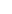Suppose that:
We buy 100 pieces from “A” coin at price of 0.00006000 BTC.
We set the selling price at 0.00006300 BTC or at 5% above of the initial investment.
Therefore the desired total profit will be 100 x 0.00000300 = 0.00030000 BTC.
Suppose now that the price drops to 0.00005400 BTC and we buy another 100 items.
The average purchase price of the currency is 0.00005700 BTC (0.00006000 + 0.00005400) / 2).
We cancel the previous selling order of 100 coins at 0.00006300 BTC and place a new one of 200 new coins as follows:
200 x 0.00005700 = 0.01140000 BTC (Cost) + 0.00030000 BTC (Profit) = 0.01170000 / 200 = 0.00005850 BTC.

If the price goes up and we sell at the above price, then we have secured the initial profitability we set as target. If the price goes down, then according to this strategy, we will have to buy more pieces of this coin. Gradually, however, if the descent of the price continues for a long time, then a serious issue of liquidity may appear. According to the logic we are following so far, the next move would be to buy 200 coins at a lower price and then 400 pieces to an even lower price. It is obvious that sooner or later our investment will grow and will deprive us of substantial capital from other investments. Below, we will see how we can deal with such situations.to signal pageImplementation ❺❺❺ - average rating 5 from 5 (based on 189 user reviews)